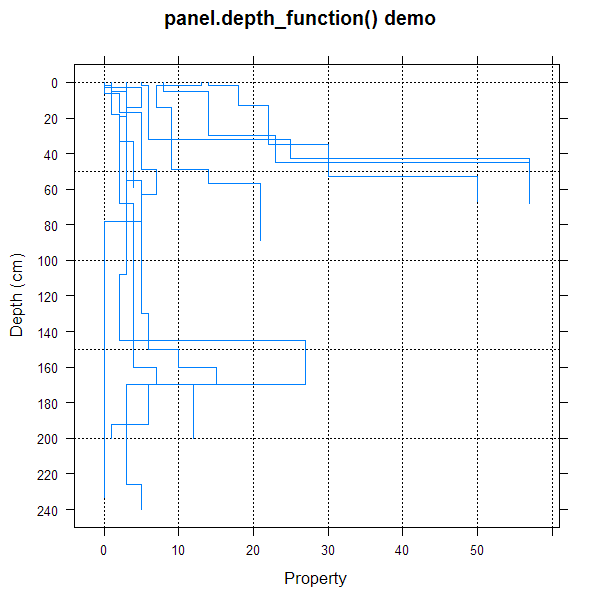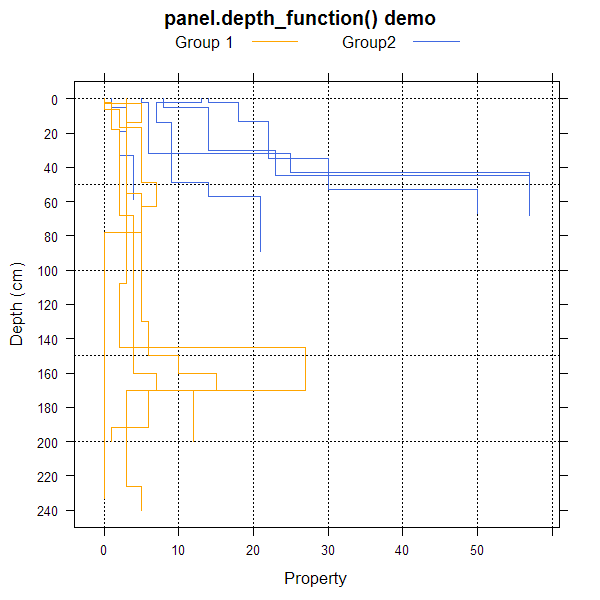panel.depth_function {aqp} R Documentation

## Lattice Panel Function for Soil Profiles

### Description

Panel function for plotting grouped soil property data, along with upper and lower estimates of uncertainty.

### Usage

```panel.depth_function(x, y, id, upper = NA, lower = NA,
subscripts = NULL, groups = NULL, sync.colors=FALSE, cf=NA,
cf.col=NA, cf.interval=20, ...)
```

### Arguments

 `x` x values (generated by calling lattice function) `y` y values (generated by calling lattice function) `id` vector of id labels, same length as x and y–only required when plotting segments (see Details section) `upper` vector of upper confidence envelope values `lower` vector of lower confidence envelope values `subscripts` paneling indices (generated by calling lattice function) `groups` grouping data (generated by calling lattice function) `sync.colors` optionally sync the fill color within the region bounded by (lower–upper) with the line colors `cf` optionally annotate contributing fraction data at regular depth intervals see `slab` `cf.col` optionall color for contributing fraction values, typically used to override the line color `cf.interval` number of depth units to space printed contributing fraction values `...` further arguments to lower-level lattice plotting functions, see below

### Details

This function can be used to replace `panel.superpose` when plotting depth function data. When requested, contributing fraction data are printed using colors the same color as corresponding depth function lines unless a single color value is given via `cf.col`.

### Author(s)

Dylan E. Beaudette

### References

http://casoilresource.lawr.ucdavis.edu/

`sp1`, `slice`, `slab`

### Examples

``````library(lattice)
data(sp1)

# 1. plotting mechanism for step-functions derived from soil profile data
xyplot(cbind(top,bottom) ~ prop, data=sp1,id=sp1\$id,
panel=panel.depth_function, ylim=c(250,-10),
scales=list(y=list(tick.number=10)), xlab='Property',
ylab='Depth (cm)', main='panel.depth_function() demo'
)
````````````# 1.1 include groups argument to leverage lattice styling framework
sp1\$group <- factor(sp1\$group, labels=c('Group 1', 'Group2'))

xyplot(cbind(top,bottom) ~ prop, groups=group, data=sp1, id=sp1\$id,
panel=panel.depth_function, ylim=c(250,-10),
scales=list(y=list(tick.number=10)), xlab='Property',
ylab='Depth (cm)', main='panel.depth_function() demo',
auto.key=list(columns=2, points=FALSE, lines=TRUE),
par.settings=list(superpose.line=list(col=c('Orange','RoyalBlue')))
)
``````[Package aqp version 1.9.1 Index]